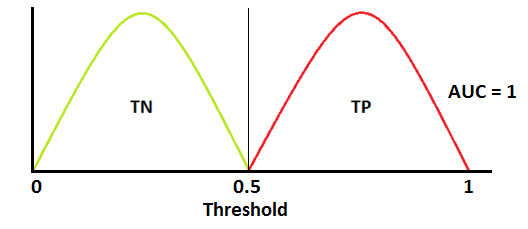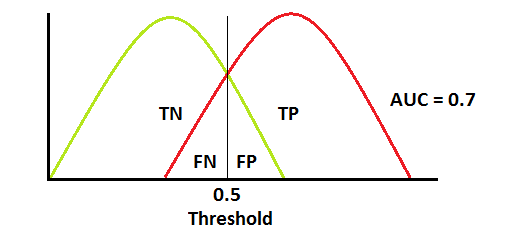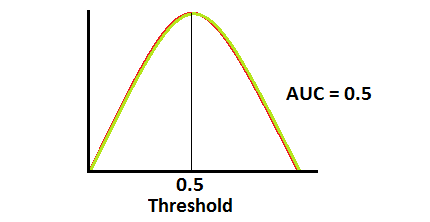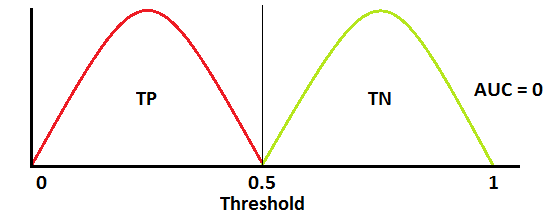# How to interpret FPR and TPR in ROC curve?

I am playing with ROC and trying to draw some curves. I am using example from this scikit page. I do not understand one thing: when I print out the content of tpr and fpr, I see two arrays of numbers (21 elems in each) - why is that?

TPR (True Positive Ratio) is a proportion of those tuples classified as positives to all real positive tuples. So, it should be one number

I see it as follow: I take classifier (like Decision Tree), train it on some data and finally test it. Then I can calculate TPR and FPR and I should have only two values.

Instead, I receive arrays. Why is that? I tried to change classifier from that example to Decision Tree and then I receive only one point (which is usually [0, 1]). How can I draw ROC curve on decision tree? Or - to be more precise with my question - why do I get an array of values as my TRP/FPR instead of single values?

If you plot your 21 pair of points $$(f_i, t_i)$$, where $$(f_i, t_i)$$ point is connected to $$(f_{i+1}, t_{i+1})$$ etc., you get the ROC curve. The fpr and tpr of model depend on the decision threshold.

For example, in a binary classification, suppose model outputs:

$$(\mbox{true label, prediction})=(c, c')=\{(1, 0.8), (1, 0.6), (1, 0.4), (0, 0.2), (0, 0.4)\}$$

for three points from class 1 and two points from class 0. Here are $$(f_i, t_i)$$ pairs for 3 decision thresholds (two arrays of size 3):

Decision threshold 1: if $$c' \geq 0.9$$, label 1, otherwise label $$0$$

points labeled 1 = $$\{\}$$
false positives = $$\{\} \rightarrow f_1=0/2=0$$
true positives = $$\{\} \rightarrow t_1=0/3 = 0$$
First ROC point = $$(f_1, t_1)=(0, 0)$$

Decision threshold 2: if $$c' \geq 0.5$$, label 1, otherwise label $$0$$

points labeled 1 = $$\{(1, 0.8), (1, 0.6)\}$$
false positives = $$\{\} \rightarrow f_1=0/2=0$$
true positives = $$\{(1, 0.8), (1, 0.6)\} \rightarrow t_1=2/3 = 0.66$$
Second ROC point = $$(f_2, t_2)=(0, 0.66)$$

Decision threshold 3: if $$c' \geq 0$$, label 1, otherwise label $$0$$

points labeled 1 = $$\{(1, 0.8), (1, 0.6), (1, 0.4), (0, 0.2), (0, 0.4)\}$$
false positives = $$\{(0, 0.2), (0, 0.4)\} \rightarrow f_1=2/2=1.0$$
true positives = $$\{(1, 0.8), (1, 0.6), (1, 0.4)\} \rightarrow t_1=3/3 = 1.0$$
Third ROC point = $$(f_3, t_3)=(1.0, 1.0)$$

ROC curve is drawn by connecting these three points as follows:

$$(0, 0) \rightarrow (0, 0.66) \rightarrow (1.0, 1.0)$$

With more fine-grained decision thresholds, we get a more accurate ROC curve.

As explained here in the docs of the function returning the ROC Curve, they return an array because when you interpret a ROC curve what you're looking at the performance of the predictor given a threshold. So, it means that, if I'm looking for only classifications that the model is above 80% sure, the ROC curve will have a different TPR/FPR than a threshold of 90%.

If you have further questions, here's a great link explaining it.

In Machine Learning, performance measurement is an essential task. So when it comes to a classification problem, we can count on an AUC - ROC Curve. AUC - ROC curve is a performance measurement for classification problem at various thresholds settings. It tells how much model is capable of distinguishing between classes.

$$TPR/Recall/Sensitivity = \frac{TP}{TP+FN}$$ $$Specificity = \frac{TN}{TN+FP}$$ $$FPR=1-Specificity = \frac{FP}{TN+FP}$$

and the model performance could be interpreted asThis is an excerpt of this article Understanding AUC - ROC Curve

because the array is actually a function who's AUC (area under the curve) is a single number that defines lets a the performance of a binary classifier using the TPR and FPR values.